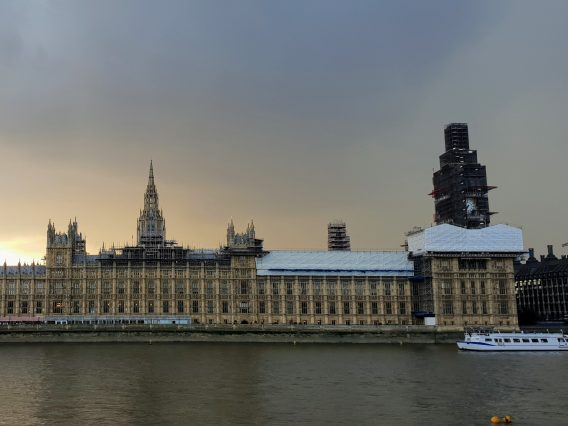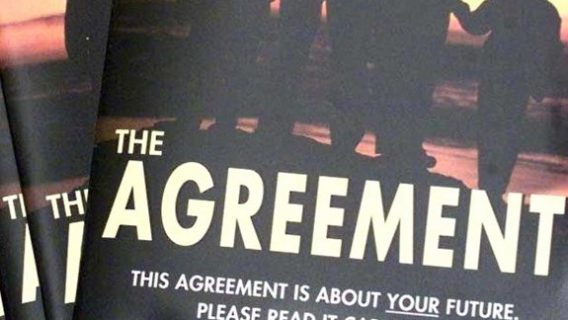• Rounding and significant places (90 KB , PDF)

Rounding is the process of reducing the number of significant digits in a number. This can help make it easier to remember and use. The result of rounding is a “shorter” number having fewer non-zero digits yet similar in magnitude. The most common uses are where numbers are “longest” – very large numbers rounded to the nearest billion, or million, or very long decimals rounded to one or two decimal places.  The result is less precise than the unrounded number.

Example: Turnout in Witney in the 2010 General Election was 73.34%. Rounded to one decimal place (nearest tenth) it is 73.3%, because 73.34 is closer to 73.3 than to 73.4.  Rounded to the nearest whole number it becomes 73% and to the nearest ten it becomes 70%.  Rounding to a larger unit tends to take the rounded number further away from the unrounded number.

The most common method used for rounding is the following:

• Decide what units you want to round to (hundreds, thousands, number of decimal places etc.) and hence which is the last digit to keep. The first example above rounds to one decimal place and hence the last digit is 3.
• Increase it by 1 if the next digit is 5 or more (known as rounding up)
• Leave it the same if the next digit is 4 or less (known as rounding down)

Further examples:

• 8.074 rounded to two decimal places (hundredths) is 8.07 (because the next digit, 4, is less than 5).
• 8.0747 rounded to two decimal places is 8.07 (the next digit, 4, is less than 5).
• 2,732 rounded to the nearest ten is 2,730 (the next digit, 2 is less than 5)
• 2,732 rounded to the nearest hundred is 2,700 (the next digit, 3, is less than 5)

For negative numbers the absolute value is rounded.

Examples:

• −6.1349 rounded to two decimal places is −6.13
• −6.1350 rounded to two decimal places is −6.14

Although it is customary to round the number 4.5 up to 5, in fact 4.5 is no nearer to 5 than it is to 4 (it is 0.5 away from either). When dealing with large sets of data, where trends are important, traditional rounding on average biases the data slightly upwards.

Another method is the round-to-even method (also known as unbiased rounding). With all rounding schemes there are two possible outcomes: increasing the rounding digit by one or leaving it alone. With traditional rounding, if the number has a value less than the half-way mark between the possible outcomes, it is rounded down; if the number has a value exactly half-way or greater than half-way between the possible outcomes, it is rounded up. The round-to-even method is the same except that numbers exactly half-way between the possible outcomes are sometimes rounded up – sometimes down. Over a large set of data the round-to-even rule tends to reduce the total rounding error, with (on average) an equal portion of numbers rounding up as rounding down. This generally reduces the upwards skewing of the result.

The round-to-even method is the following:

• Decide which is the last digit to keep.
• Increase it by 1 if the next digit is 6 or more, or a 5 followed by one or more non-zero digits.
• Leave it the same if the next digit is 4 or less
• Otherwise, all that follows the last digit is a 5 and possibly trailing zeroes; then change the last digit to the nearest even digit. That is, increase the rounded digit if it is currently odd; leave it if it is already even.

Examples:

• 8.016 rounded to two decimal places is 8.02 (the next digit (6) is 6 or more)
• 8.015 rounded to two decimal places is 8.02 (the next digit is 5, and the previous digit (1) is odd)
• 8.045 rounded to two decimal places is 8.04 (the next digit is 5, and the previous digit (4) is even)
• 8.04501 rounded to two decimal places is 8.05 (the next digit is 5, but it is followed by non-zero digits)

The process of rounding results in a less precise figure. This explains why rounded percentage totals do not always add up to 100 and why rounded absolute numbers do not always sum to the total.

Rounding to n significant places is a form of rounding by handling numbers of different scales in a uniform way. This gives a rule to where numbers are rounded to. A digit that is closer to the start of a number is “larger” and therefore considered more significant. The number of significant places tell us where to start rounding, from then on the rules are the same.

Examples. Rounding to 2 significant figures:

• 15,420 becomes 15,000 (the 1 and the 5 are the first two significant figures and the next digit, 4, is less than 5)
• 0.001586 becomes 0.0016
• 0.5 becomes 0.50 (the trailing zero indicates rounding to 2 significant figures)
• 0.07051 becomes 0.071
• 88,920 becomes 89,000

Rounding to 3 significant figures

• 15,420 becomes 15,400 (the 1, 5 and 4 are the first two significant figures and the next digit, 2, is less than 5)
• 0.001586 becomes 0.00159
• 0.5 becomes 0.500 (the trailing zero indicates rounding to 3 significant figures)
• 0.07051 becomes 0.0705
• 88,920 becomes 88,900

Other statistical literacy guides in this series:

• Rounding and significant places (90 KB , PDF)

## Related posts

• ### General debate on the 25th anniversary of the Belfast/Good Friday agreement

On Thursday 30 March there will be a general debate on the 25th anniversary of the Belfast/Good Friday agreement• ### The Data Protection and Digital Information (No. 2) Bill 2022-23

The Data Protection and Digital Information (No.2) Bill was introduced in the House of Commons on 8 March 2023.• ### The Belfast/Good Friday Agreement – 25 years on

A briefing paper on the 25th anniversary of the Belfast/Good Friday Agreement, which established power-sharing institutions in Northern Ireland.